Win up to 100% scholarship on Aakash BYJU'S JEE/NEET courses with ABNAT Win up to 100% scholarship on Aakash BYJU'S JEE/NEET courses with ABNAT

# JEE Main 2022 June 26 – Shift 2 Physics Question Paper with Solutions

The JEE Main 2022 June 26 – Shift 2 Physics Question Paper with Solutions is available here for students’ reference. The physics curriculum for Classes XI and XII is the basis for the JEE question paper. Candidates can access the question paper in PDF format on this page. Students can also use the PDF file for future reference. These solutions were written by the JEE specialists of BYJU’S. Practising the JEE Main 2022 Question Papers will help candidates raise their JEE scores. Additionally, the applicant can efficiently prepare for the upcoming exam by using this JEE Main 2022 answer key as a guide.

## JEE Main 2022 26th June Evening Shift Physics Question Paper and Solutions

#### SECTION – A

Multiple Choice Questions: This section contains 20 multiple choice questions. Each question has 4 choices (1), (2), (3) and (4), out of which ONLY ONE is correct.

1. The dimension of mutual inductance is :

(A) [ML2T–2A–1]

(B) [ML2T–3A–1]

(C) [ML2T–2A–2]

(D) [ML2T–3A–2]

Sol.

$$\begin{array}{l}\because U=\frac{1}{2}Mi^2\end{array}$$
$$\begin{array}{l}\Rightarrow [M]=\frac{[U]}{[i^2]}=\frac{ML^2T^{-2}}{A^2}\end{array}$$

= [ML2T–2A–2]

2. In the arrangement shown in figure a1, a2, a3 and a4 are the accelerations of masses m1, m2, m3 and m4 respectively. Which of the following relation is true for this arrangement?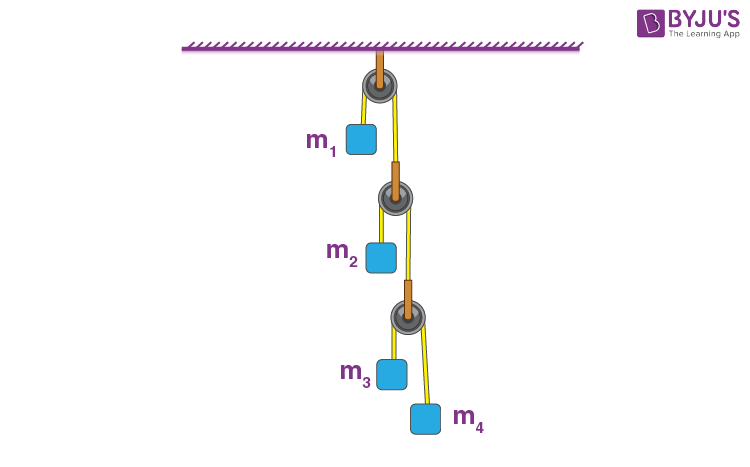(A) 4a1 + 2a2 + a3 + a4 = 0

(B) a1 + 4a2 + 3a3 + a4 = 0

(C) a1 + 4a2 + 3a3 + 2a4 = 0

(D) 2a1 + 2a2 + 3a3 + a4 = 0

Sol. From virtual work done method,

4T × a1 + 2T × a2 + T × a3 + T × a4 = 0

⇒ 4a1 + 2a2 + a3 + a4 = 0

3. Arrange the four graphs in descending order of total work done; where W1, W2, W3 and W4 are the work done corresponding to figure a, b, c and d respectively.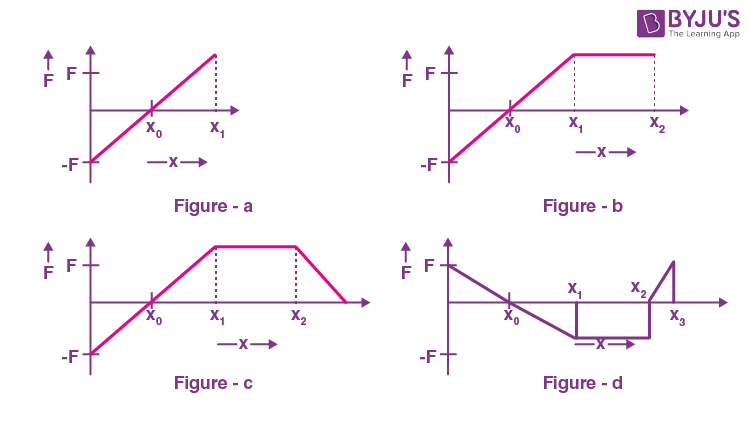(A) W3 > W2 > W1 > W4

(B) W3 > W2 > W4 > W1

(C) W2 > W3 > W4 > W1

(D) W2 > W3 > W1 > W4

Sol. Wa = 0, Wb = +ve, Wc = +ve > Wb, Wd = –ve

Wc > Wb > Wa > Wd

W3 > W2 > W1 > W4

4. A solid spherical ball is rolling on a frictionless horizontal plane surface about its axis of symmetry. The ratio of rotational kinetic energy of the ball to its total kinetic energy is –

(A) ⅖

(B) 2/7

(C) ⅕

(D) 7/10

Sol.

$$\begin{array}{l}\textup{KE}_R=\frac{1}{2}l\omega ^2\end{array}$$
$$\begin{array}{l}=\frac{1}{2}\times\frac{2}{5}\times\omega ^2\times(mR^2)\end{array}$$
$$\begin{array}{l}\textup{KE}_{total}=\frac{1}{2}\times\frac{7}{5}\times mR^2\times \omega^2\end{array}$$
$$\begin{array}{l}\therefore \frac{KE_R}{KE_{total}}=\frac{2}{7}\end{array}$$

5. Given below are two statements: One is labelled as Assertion A and the other is labelled as Reason R.

Assertion A: If we move from poles to equator, the direction of acceleration due to gravity of earth always points towards the center of earth without any variation in its magnitude.

Reason R: At equator, the direction of acceleration due to the gravity is towards the center of earth.

In the light of above statements, choose the correct answer from the options given below

(A) Both A and R are true and R is the correct explanation of A.

(B) Both A and R are true but R is NOT the correct explanation of A.

(C) A is true but R is false.

(D) A is false but R is true.

Sol. g′ = g0 – ω2Rcos2θ

θ = latitude.

6. If ρ is the density and η is coefficient of viscosity of fluid which flows with a speed v in the pipe of diameter d, the correct formula for Reynolds number Re is:

$$\begin{array}{l}(A)\ R_e=\frac{\eta d}{\rho v}\end{array}$$
$$\begin{array}{l}(B)\ R_e=\frac{\rho v}{\eta d}\end{array}$$
$$\begin{array}{l}(C)\ R_e=\frac{\rho vd}{\eta }\end{array}$$
$$\begin{array}{l}(D)\ R_e=\frac{\eta}{\rho vd}\end{array}$$

Sol.

$$\begin{array}{l}R_e=\frac{\rho vd}{\eta}\end{array}$$

Direct formula based.

7. A flask contains argon and oxygen in the ratio of 3 : 2 in mass and the mixture is kept at 27°C. The ratio of their average kinetic energy per molecule respectively will be

(A) 3 : 2

(B) 9 : 4

(C) 2 : 3

(D) 1 : 1

Sol.

$$\begin{array}{l}\textup{KE}_{avg}=\frac{3}{2}kT\end{array}$$
(At lower temperature)

As temperature is same for both the gases.

⇒ Both gases will have same average kinetic energy.

$$\begin{array}{l}\Rightarrow \frac{(\textup{KE}_{avg})_{argon}}{(\textup{KE}_{avg})_{oxygen}}=\frac{1}{1}\end{array}$$

8. The charge on capacitor of capacitance 15 μF in the figure given below is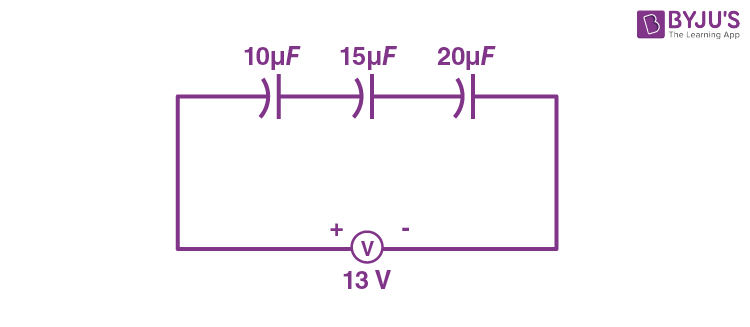(A) 60 μC

(B) 130 μC

(C) 260 μC

(D) 585 μC

Sol.

$$\begin{array}{l}C_{eq}=\frac{120}{26}~\mu \textup{F}\end{array}$$
$$\begin{array}{l}\Rightarrow Q_{flown}\ \text{or}\ Q=\frac{13\times120}{26}~\mu C=60~\mu \textup{C}\end{array}$$

⇒ Charge on 15 μF capacitor = 60 μC

As all the capacitors are in series.

9. A parallel plate capacitor with plate area A and plate separation d = 2 m has a capacitance of 4 μF. The new capacitance of the system if half of the space between them is filled with a dielectric material of dielectric constant K = 3 (as shown in figure) will be :(A) 2 μF

(B) 32 μF

(C) 6 μF

(D) 8 μF

Sol. Equivalent circuit is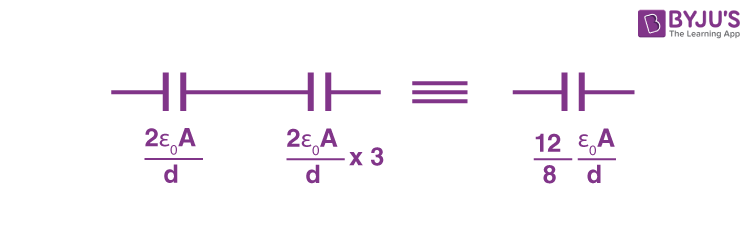Now

$$\begin{array}{l}\frac{\epsilon _0A}{d}=4\mu \textup{F}\end{array}$$
$$\begin{array}{l}\Rightarrow \frac{12}{8}\frac{\epsilon _0A}{d}=6\mu\textup{F}\end{array}$$

10. Sixty four conducting drops each of radius 0.02 m and each carrying a charge of 5 μC are combined to form a bigger drop. The ratio of surface density of bigger drop to the smaller drop will be:

(A) 1 : 4

(B) 4 : 1

(C) 1 : 8

(D) 8 : 1

Sol. q′ = 64q … (i)

A′ = 16 A … (ii)

Dividing (i) & (ii),

σ′ = 4σ

$$\begin{array}{l}\Rightarrow \frac{\sigma ‘}{\sigma }=\frac{4}{1}\end{array}$$

11. The equivalent resistance between points A and B in the given network is: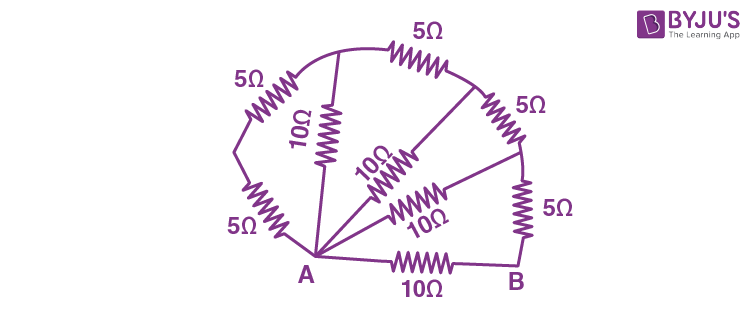(A) 65 Ω

(B) 20 Ω

(C) 5 Ω

(D) 2 Ω

Sol. Initially 5 Ω and 5 Ω are in series and then in parallel with 10 Ω this pattern continues thus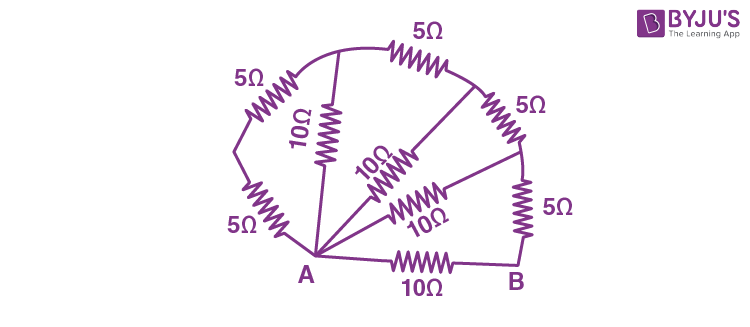Rnet = 5 Ω

12. A bar magnetic having a magnetic moment of 2.0 × 105 JT–1, is placed along the direction of uniform magnetic field of magnitude B = 14 × 10–5 T. The work done in rotating the magnet slowly through 60° from the direction of field is:

(A) 14 J

(B) 8.4 J

(C) 4 J

(D) 1.4 J

Sol.

$$\begin{array}{l}U=-\vec{M}.\vec{B}\end{array}$$

So UfUi = –MB(1 – cosθ)

= – 14J

So W = – ΔU = 14J

13. Two coils of self inductance L1 and L2 are connected in series combination having mutual inductance of the coils as M. The equivalent self inductance of the combination will be:$$\begin{array}{l}(A)\ \frac{1}{L_1} + \frac{1}{L_2} + \frac{1}{M}\end{array}$$

(B) L1 + L2 + M

(C) L1 + L2 + 2M

(D) L1 + L2 – 2M

Sol. Self inductances are in series but their mutual inductances are linked oppositely so equivalent self inductance

L = L1 + L2MM = L1 + L2 – 2M

14. A metallic conductor of length 1 m rotates in a vertical plane parallel to east-west direction about one of its end with angular velocity 5 rad s–1. If the horizontal component of earth’s magnetic field is 0.2 × 10–4 T, then emf induced between the two ends of the conductor is:

(A) 5 μV

(B) 50 μV

(C) 5 mV

(D) 50 mV

Sol. Emf = (½)Bωl2

= (½)× 0.2 × 10–4 × 5 × 12 V

= 0.5 × 10–4 V

= 50 μV

15. Which is the correct ascending order of wavelengths?

(A) λvisible < λX-ray < λgamma-raymicrowave

(B) λgamma-ray < λX-ray < λvisible < λmicrowave

(C) λX-ray < λgamma-ray < λvisible < λmicrowave

(D) λmicrowave < λvisible < λgamma-ray < λX-ray

Sol. Wave length of microwave is maximum then visible light then X-rays and then gamma rays so the correct order will be

λgamma-ray < λX-ray < λvisible < λmicrowave

16. For a specific wavelength 670 nm of light from a galaxy moving with velocity ν, the observed wavelength is 670.7 nm. The value of ν is:

(A) 3 × 108 ms–1

(B) 3 × 1010 ms–1

(C) 3.13 × 105 ms–1

(D) 4.48 × 105 ms–1

Sol.

$$\begin{array}{l}\lambda _{obs}=\lambda _{source}\sqrt{\frac{1+\frac{\nu }{C}}{1-\frac{\nu }{C}}} \end{array}$$

For ν << C,

$$\begin{array}{l}\frac{670.7}{670}=1+\frac{\nu }{C} \end{array}$$
$$\begin{array}{l}\Rightarrow v=\frac{0.7}{670}\times3\times10^8~\textup{m/s}\end{array}$$
$$\begin{array}{l}\Rightarrow v\simeq 3.13\times10^5~\textup{m/s}\end{array}$$

17. A metal surface is illuminated by a radiation of wavelength 4500 Å. The rejected photo-electron enters a constant magnetic field of 2 mT making an angle of 90° with the magnetic field. If it starts revolving in a circular path of radius 2 mm, the work function of the metal is approximately:

(A) 1.36 eV

(B) 1.69 eV

(C) 2.78 eV

(D) 2.23 eV

Sol.

$$\begin{array}{l}\frac{hc}{\lambda}-\phi =KE………(i)\end{array}$$
$$\begin{array}{l}R=\frac{mv}{Bq}=\frac{\sqrt{2m(KE)}}{Bq}………(ii)\end{array}$$

Putting the values,

$$\begin{array}{l}\phi\simeq 1.36\ \text{eV} \end{array}$$

18. A radioactive nucleus can decay by two different processes. Half-life for the first process is 3.0 hours while it is 4.5 hours for the second process. The effective half-life of the nucleus will be:

(A) 3.75 hours

(B) 0.56 hours

(C) 0.26 hours

(D) 1.80 hours

Sol.$$\begin{array}{l}\frac{dA}{dt}-(-\lambda _1A)+(-\lambda _2A)\end{array}$$
$$\begin{array}{l}\Rightarrow \frac{dA}{dt}=-(\lambda _1+\lambda _2)A\end{array}$$

⇒ λeff = λ1 + λ2

$$\begin{array}{l}\Rightarrow \frac{ln2}{(t_{1/2})_{eff}}=\frac{ln2}{(t_{1/2})_{1}}+\frac{ln2}{(t_{1/2})_{2}}\end{array}$$
$$\begin{array}{l}\Rightarrow (t_{1/2})_{eff}=\frac{4.5\times3}{7.5}~\textup{hours}=1.8~\textup{hours}\end{array}$$

19. The positive feedback is required by an amplifier to act an oscillator. The feedback here means:

(A) External input is necessary to sustain ac signal in output

(B) A portion of the output power is returned back to the input

(C) Feedback can be achieved by LR network

(D) The base-collector junction must be forward biased

Sol. Feedback means a portion of the output power is fed to the inputs.

20. A sinusoidal wave y(t) = 40sin(10 × 106 πt) is amplitude modulated by another sinusoidal wave x(t) = 20sin(1000πt). The amplitude of minimum frequency component of modulated signal is:

(A) 0.5

(B) 0.25

(C) 20

(D) 10

Sol.

Modulate signal s(t) ≡ [1 + 20sin(1000πt)]sin(107πt)

≡ sin(107πt) + 10cos(107πt – 103πt) + 10cos(107πt + 103πt)

⇒ Required amplitude = 10

#### SECTION – B

Numerical Value Type Questions: This section contains 10 questions. In Section B, attempt any five questions out of 10. The answer to each question is a NUMERICAL VALUE. For each question, enter the correct numerical value (in decimal notation, truncated/rounded-off to the second decimal place; e.g. 06.25, 07.00, –00.33, –00.30, 30.27, –27.30) using the mouse and the on-screen virtual numeric keypad in the place designated to enter the answer.

1. A ball is projected vertically upward with an initial velocity of 50 ms–1 at t = 0 s. At t = 2 s, another ball is projected vertically upward with same velocity. At t = _____s, second ball will meet the first ball.

(g = 10 ms–2)

Sol At t = 2 s, v1 = 50 – 2 × 10 = 30 m/s

v2 = v2

arel = gg = 0

$$\begin{array}{l}S=\frac{u^2-v^2}{2g}=\frac{50^2-30^2}{2\times10}=\frac{1600}{20}=80~\textup{m}\end{array}$$

vrel = 50 – 30 = 20 m/s

$$\begin{array}{l}\therefore \Delta t=\frac{80}{20}=4\textup{s} \end{array}$$

∴ Required time t = 2 + 4 = 6 s

2. A batsman hits back a ball of mass 0.4 kg straight in the direction of the bowler without changing its initial speed of 15 ms–1. The impulse imparted to the ball is _________ Ns.

Sol. I = mΔv

= 0.4 × 2 × 15 = 12 Ns

3. A system to 10 balls each of mass 2 kg are connected via massless and unstretchable string. The system is allowed to slip over the edge of a smooth table as shown in figure. Tension on the string between the 7th and 8th ball is ______ N when 6th ball just leaves the table.Sol. At given instant

$$\begin{array}{l}a_{sys}=\frac{6 m\times g}{10m}=\frac{6g}{10}\end{array}$$

T78 = (3m) × asys

$$\begin{array}{l}=(3m)\times\left(\frac{6g}{10}\right)\end{array}$$
$$\begin{array}{l}=\frac{3\times 2\times 6\times 10}{10}=36~\textup{N}\end{array}$$

4. A geyser heats water flowing at a rate of 2.0 kg per minute from 30°C to 70°C. If geyser operates on a gas burner, the rate of combustion of fuel will be __________ g min–1.

[Heat of combustion = 8 × 103 Jg–1, Specific heat of water = 4.2 Jg–1 °C–1]

Sol. Q = msΔT

$$\begin{array}{l}\frac{dQ}{dt}=\left(\frac{dm}{dt}\right)_{water}S\Delta T=\left(\frac{dm}{dt}\right)_{oil}C\end{array}$$
$$\begin{array}{l}\Rightarrow 2\times 4.2\times 10^3\times 40=\left(\frac{dm}{dt}\right)_{oil}\times 8\times 10^6\end{array}$$
$$\begin{array}{l}\Rightarrow \left(\frac{dm}{dt}\right)_{oil}=\frac{8\times 4.2\times 10^4}{8\times 10^6}\textup{kg/minute}\end{array}$$

= 42 g/minute

5. A heat engine operates with the cold reservoir at temperature 324 K. The minimum temperature of the hot reservoir, if the heat engine takes 300 J heat from the hot reservoir and delivers 180 J heat to the cold reservoir per cycle, is _____ K.

Sol.

$$\begin{array}{l}\left(1-\frac{324}{T_H}\right)=\frac{300-180}{300}\end{array}$$
$$\begin{array}{l}1-\frac{2}{5}=\frac{324}{T_H}\end{array}$$
$$\begin{array}{l}T_H=\frac{324\times 5}{3}=540\end{array}$$

6. A set of 20 tuning forks is arranged in a series of increasing frequencies. If each fork gives 4 beats with respect to the preceding fork and the frequency of the last fork is twice the frequency of the first, then the frequency of last fork is _______Hz.

Sol. Given ν20 = 2ν1

Alsoν20 = 4 × 19 + ν1

Soν20 = 152 Hz

7. Two 10 cm long, straight wires, each carrying a current of 5 A are kept parallel to each other. If each wire experienced a force of 10–5 N, then separation between the wires is _____ cm.

Sol.

$$\begin{array}{l}\frac{dF}{dl}=\frac{\mu _0i_1i_2}{2\pi d}\end{array}$$

So

$$\begin{array}{l}\frac{2\times 10^{-7}\times 5\times 5}{d}=\frac{10^{-5}}{10\times 10^{-2}}\end{array}$$
$$\begin{array}{l}d=\frac{2\times 10^{-7}\times 5\times 5}{10^{-4}}\end{array}$$

= 50 mm

= 5 cm

8. A small bulb is placed at the bottom of a tank containing water to a depth of √7 m. The refractive index of water is 4/3. The area of the surface of water through which light from the bulb can emerge out is xπ m2. The value of x is ________.

Sol.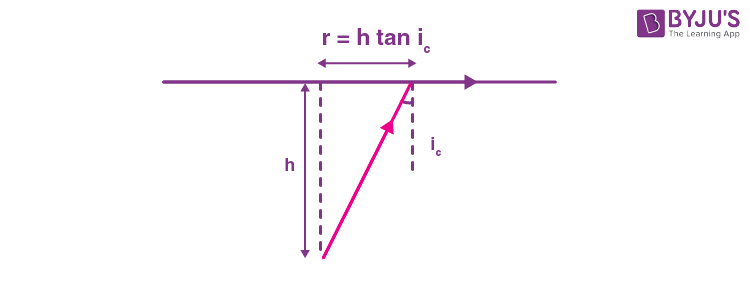So

$$\begin{array}{l}r=h\frac{sin~i_c}{\sqrt{1-sin^2~i_c}}\end{array}$$

So A = πr2

$$\begin{array}{l}=\frac{\pi h^2sin^2~i_c}{1-sin^2~i_c}\end{array}$$
$$\begin{array}{l}=\frac{\pi 7\times \frac{9}{16}}{1-\frac{9}{16}}=\frac{\pi \times 7\times 9}{7}=9\pi\end{array}$$

9. A travelling microscope is used to determine the refractive index of a glass slab. If 40 divisions are there in 1 cm on main scale and 50 Vernier scale divisions are equal to 49 main scale divisions, then least count of the travelling microscope is _______× 10–6 m.

Sol. 40 M = 1 cm

⇒ M = 0.025 cm ………..(1)

Also, 50 V = 49 M

⇒ Least count = M – V

$$\begin{array}{l}=M-\frac{49}{50}M\end{array}$$
$$\begin{array}{l}=\frac{M}{50}\end{array}$$
$$\begin{array}{l}\Rightarrow \textup{LC}=\frac{0.025}{50}~\textup{cm}\end{array}$$
$$\begin{array}{l}=\frac{250}{50}\times10^{-6}~\textup{m}\end{array}$$

⇒ LC = 5 × 10–6 m

10. The stopping potential for photoelectrons emitted from a surface illuminated by light of wavelength 6630 Å is 0.42 V. If the threshold frequency is x × 1013/s, where x is ____ (nearest integer).

(Given, speed of light = 3 × 108 m/s, Planck’s constant = 6.63 × 10–34 Js)

Sol.

$$\begin{array}{l}\frac{hc}{\lambda}-\phi =\textup{KE}=eV_0\end{array}$$
$$\begin{array}{l}\Rightarrow \frac{6.63\times 10^{-34}\times 3\times 10^8}{6630\times 10^{-10}}-6.63\times 10^{-34}f_{th}=1.6\times 10^{-19}\times 0.4\end{array}$$

fth ≃35.11 × 1013 H

### Download PDF of JEE Main 2022 June 26 Shift 2 Physics Paper & Solutions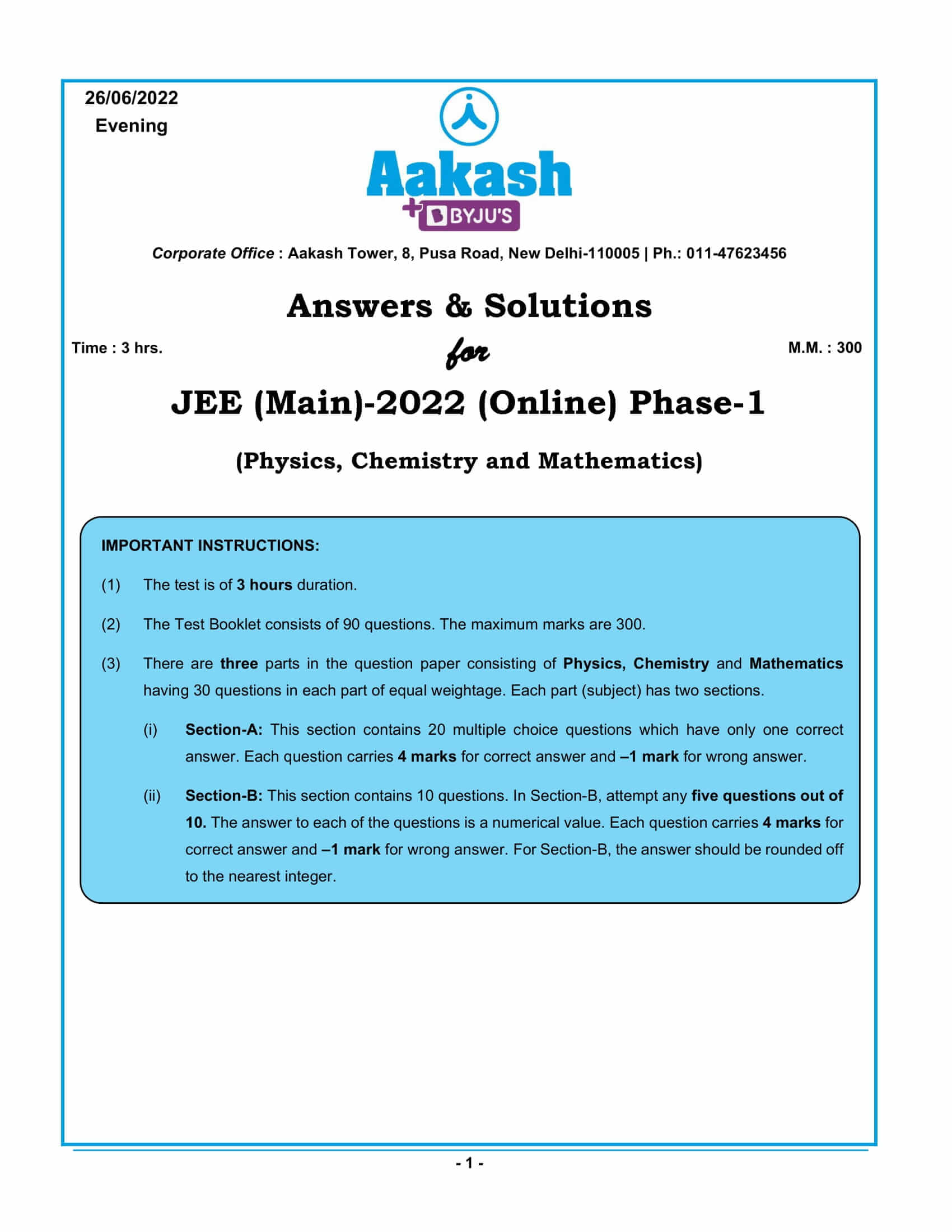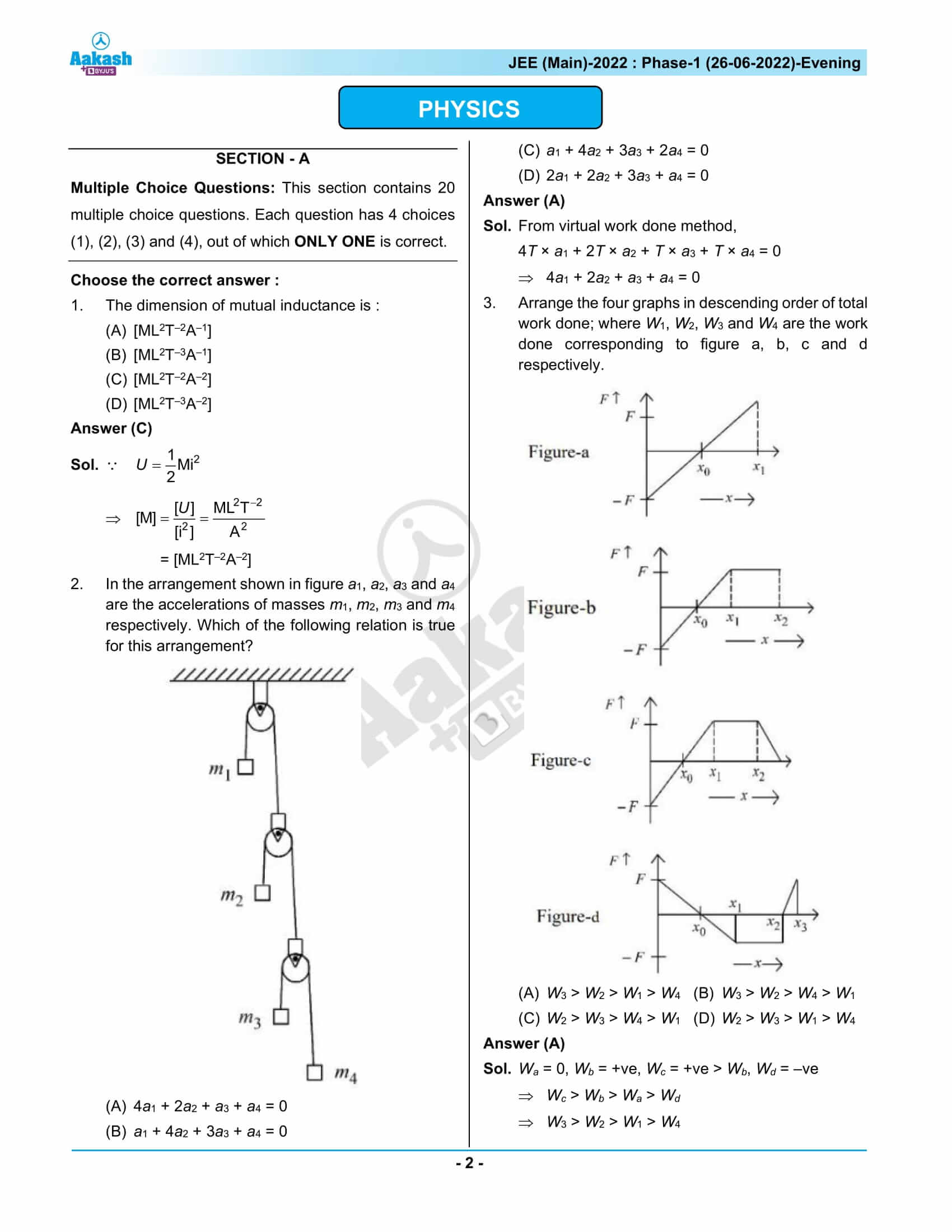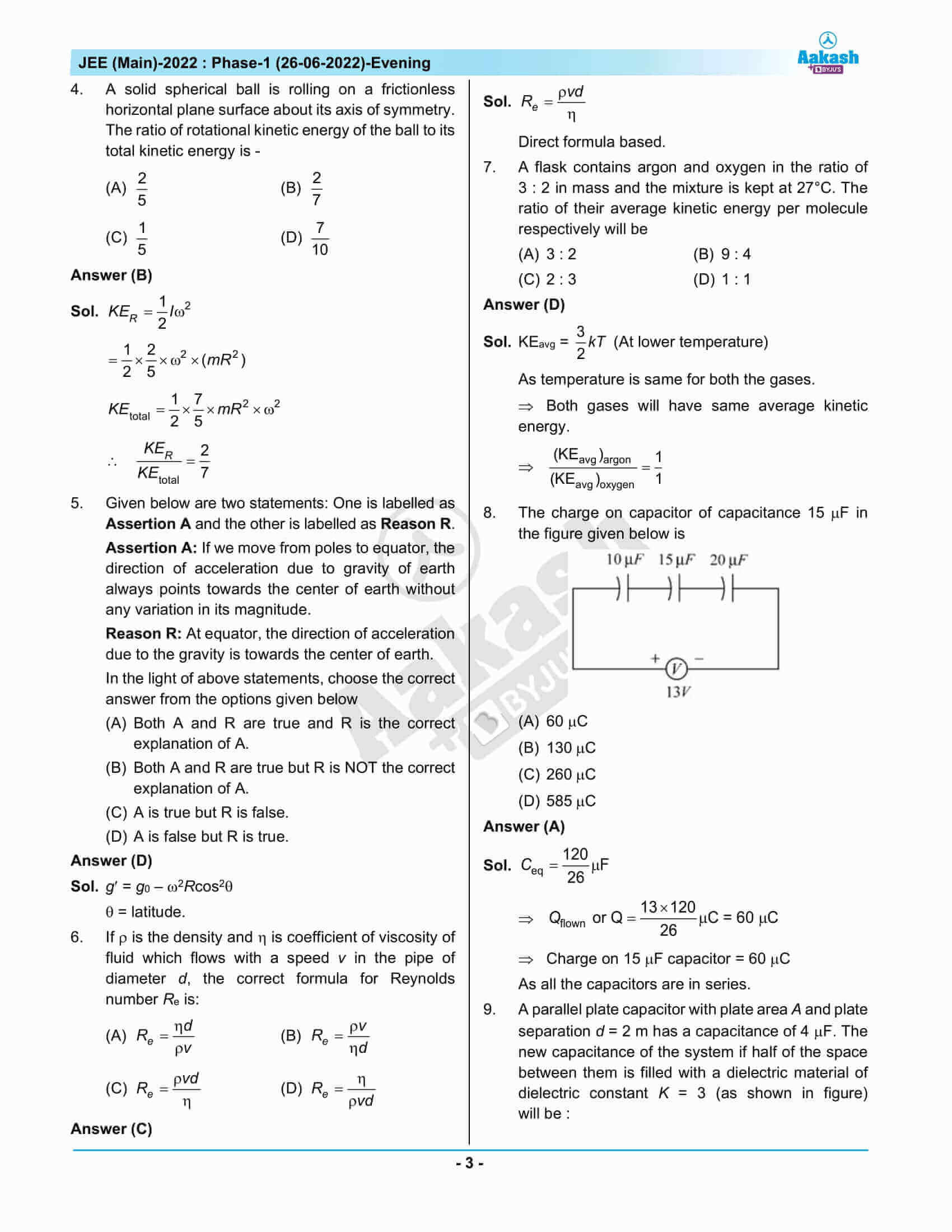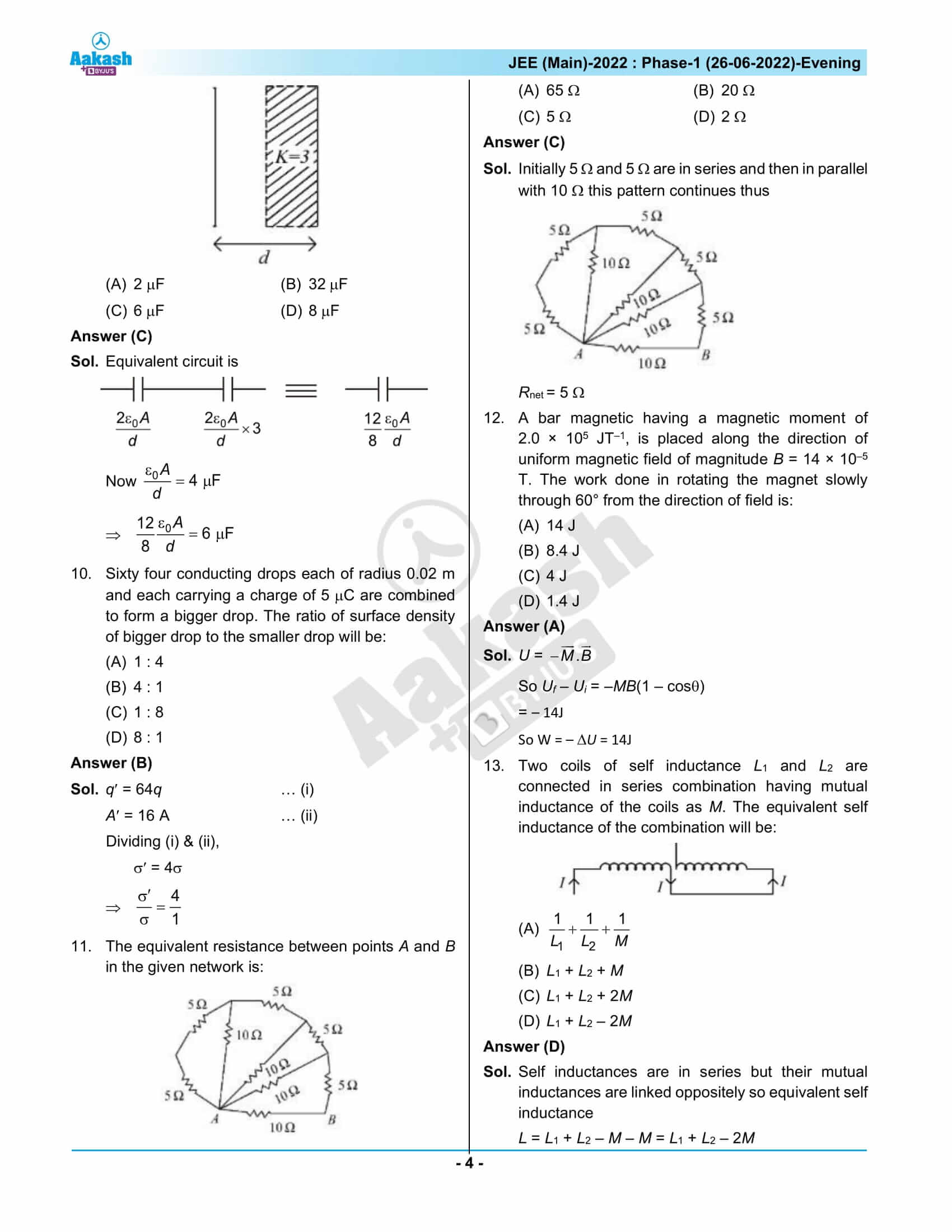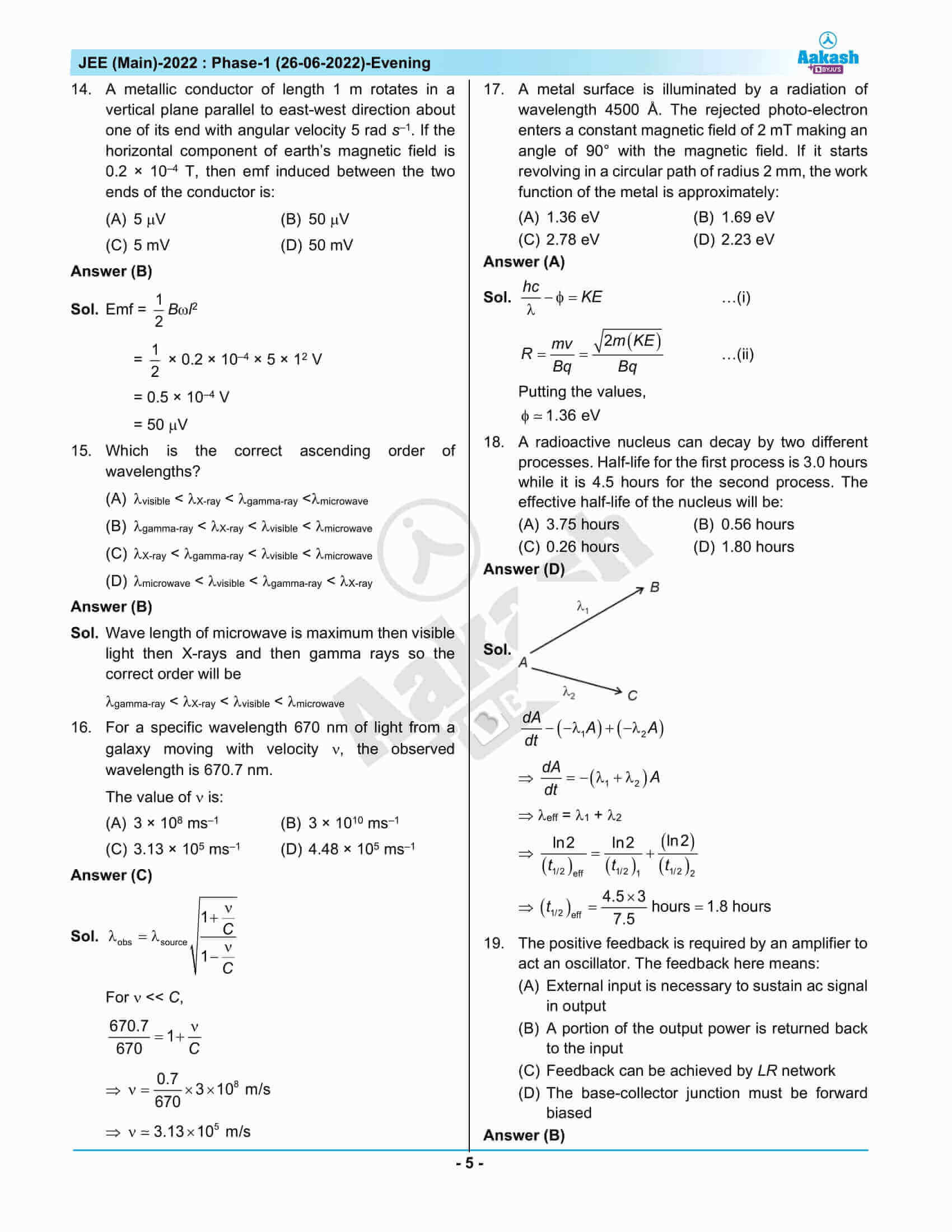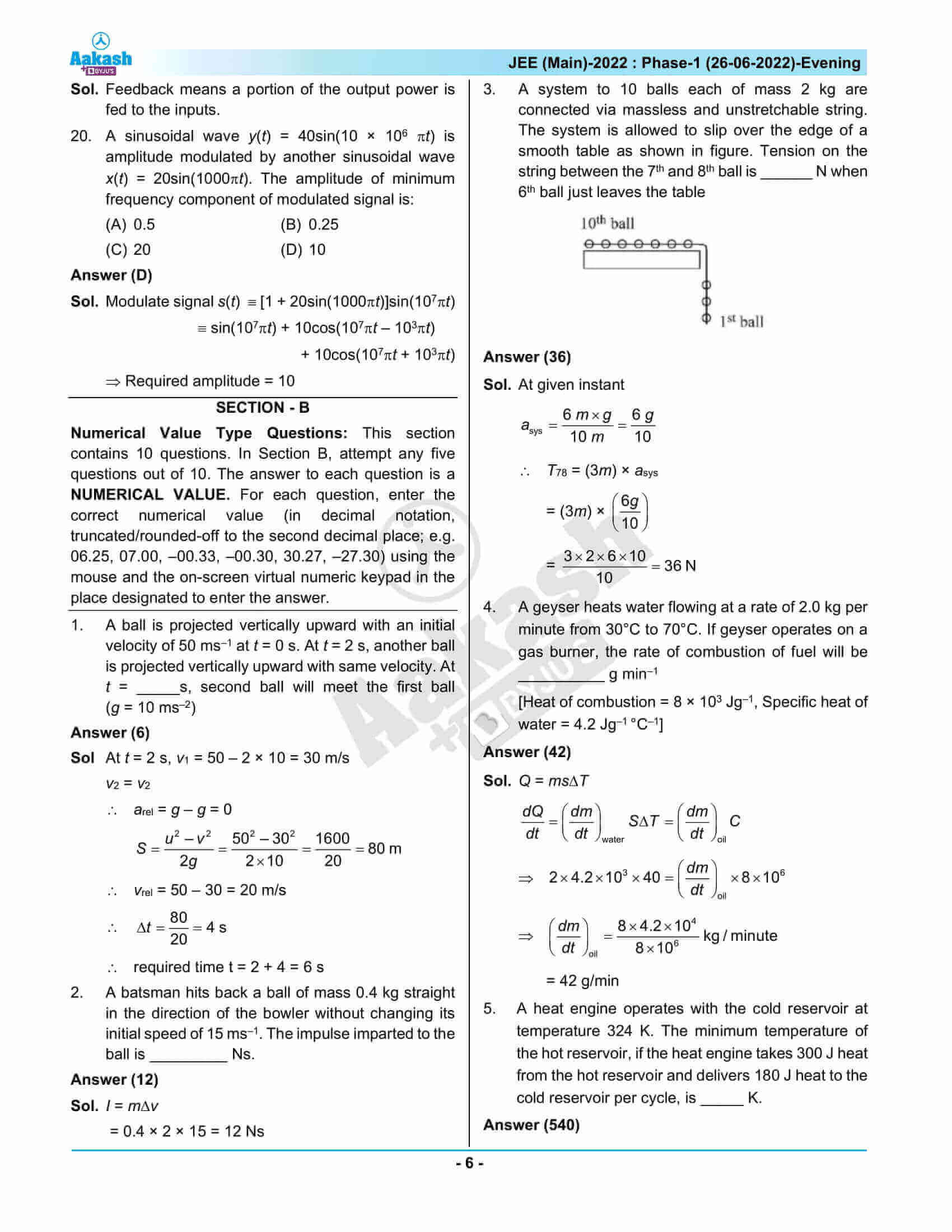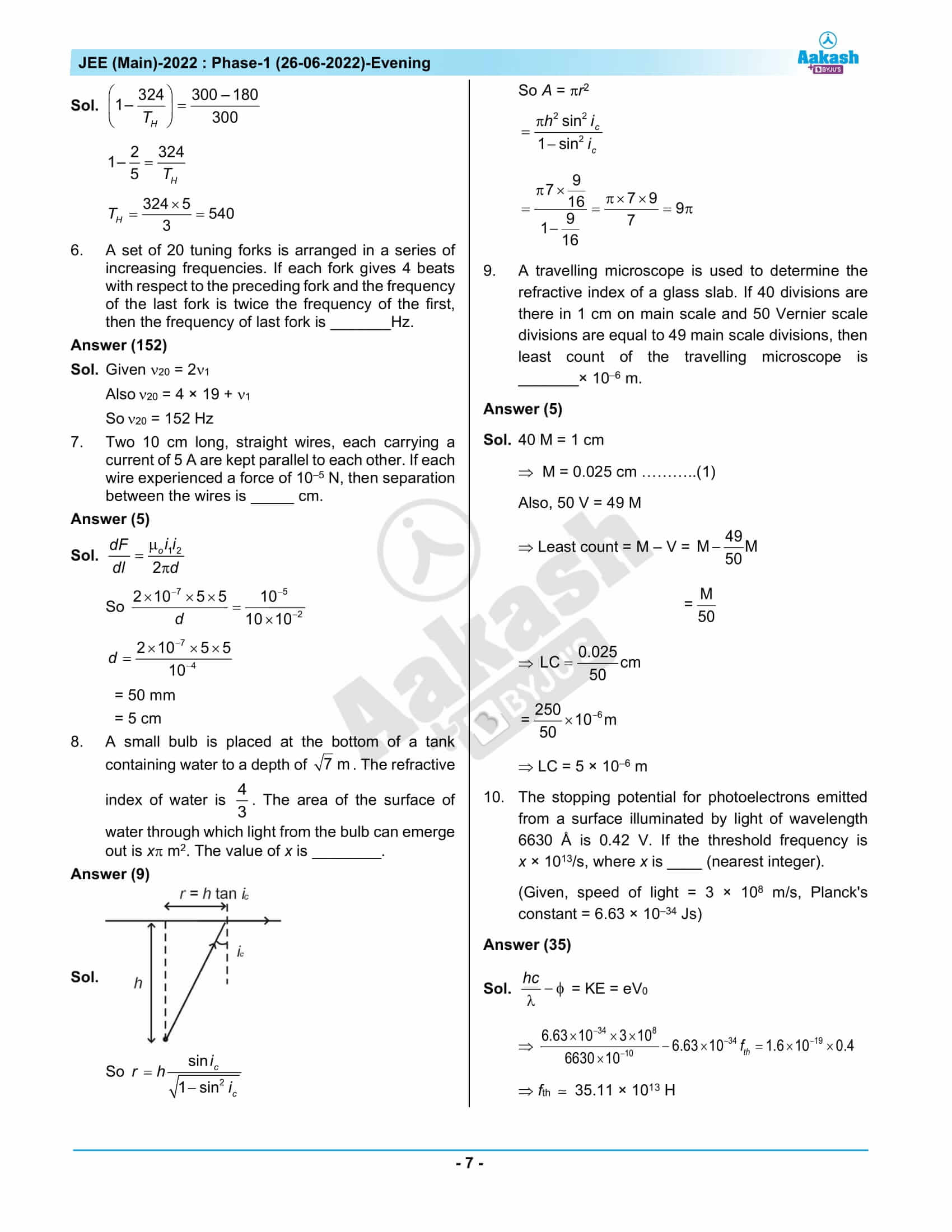## JEE Main 2022 June 26th Shift 2 Question Paper – Solutions

### JEE Main 2022 June 26 Shift 2 Question Paper – Physics Solutions### JEE Main 2022 June 26 Shift 2 Question Paper – Chemistry Solutions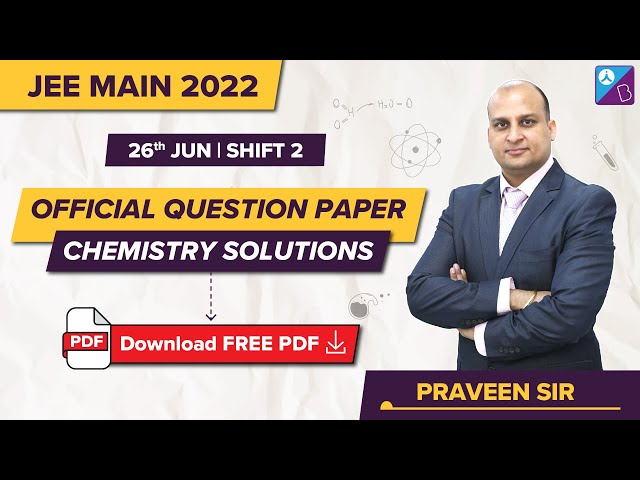### JEE Main 2022 June 26 Shift 2 Question Paper – Maths Solutions## Frequently Asked Questions – FAQs

Q1

### Were Physics questions easier compared to Chemistry and Mathematics questions in JEE Main 2022 June 26th shift 2 question paper?

Compared to Mathematics and Chemistry, Physics questions were easy. There were 10 easy questions in Physics, 12 questions of medium difficulty, and 1 difficult question of the 23 memory-based questions.

Q2

### What was the difficulty level of Physics Questions on a scale of 1 to 3?

The difficulty level of the Physics questions was rated 1.61 on a scale of 1 to 3.

Q3

### What are the Class 11 Physics chapters included in JEE Main syllabus?

Few of the Physics chapters from the Class 11 curriculum included for JEE Main are
Units and Measurements
System of Particles and Rotational Motion
Mechanical Properties of Fluids
Motion In A Straight Line
Work, Energy and Power
Gravitation
Laws of Motion
Waves

Q4

### What was the class-wise question distribution of JEE Main 2022 June 26 shift 2 question paper?

Out of the 23 memory-based questions, 9 are from the Class 11 syllabus and 14 are from the Class 12 syllabus.

Q5

### What was the topic asked from the topic Electrostatic Potential and Capacitance?

The topic included in the chapter Electrostatic Potential and Capacitance is Grouping of Capacitors.